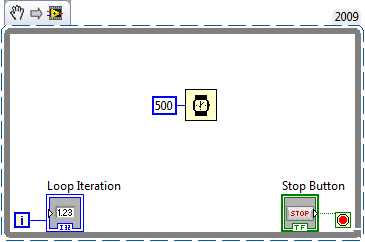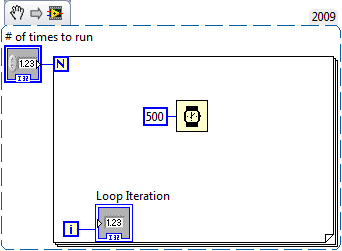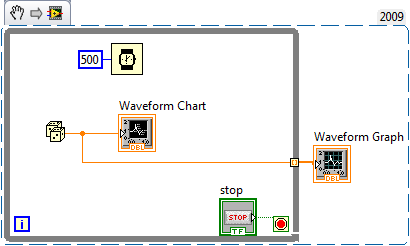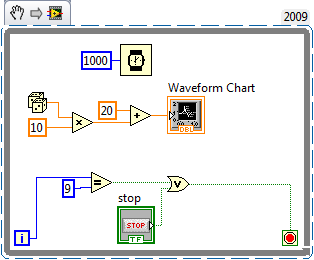# Curriculum and Labs for Engineering Education

cancel
Showing results for
Did you mean:

## Unit 2 - Fundamentals: Lesson 2

Introduction

In this lesson we are going to be talking about while loops, for loops, charts and graphs.

While loops and for loops allow us to reiterate sections of our code a set number of times or until a condition is met. Charts and graphs mean while allow us to display data in an organized manner.

Lesson Video 1

Example SnippetLesson Video 2

Example SnippetLesson Video 3

Example SnippetPractice Problem

Procedure: Create the required VI(s) to achieve the following tasks.

Requirements:

1. Create a VI which runs exactly 10 times or until a stop button is pushed
2. At 1s intervals, it calculates a random number between 20 and 30.
3. Display the random number on a waveform chart.

Practice SolutionNext Step

Unit 2 - Fundamentals: Lesson 3